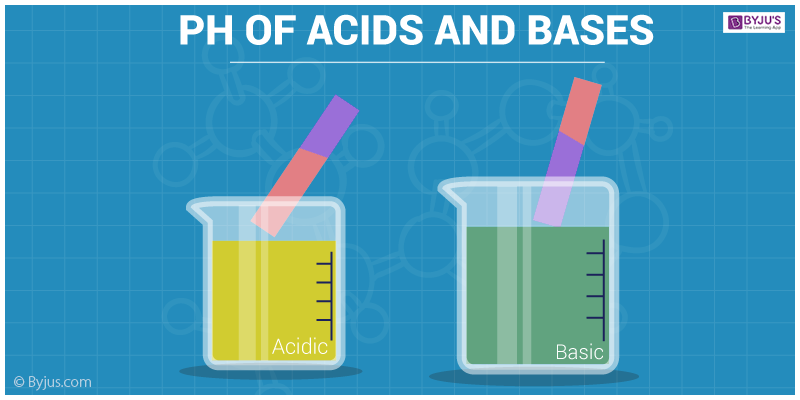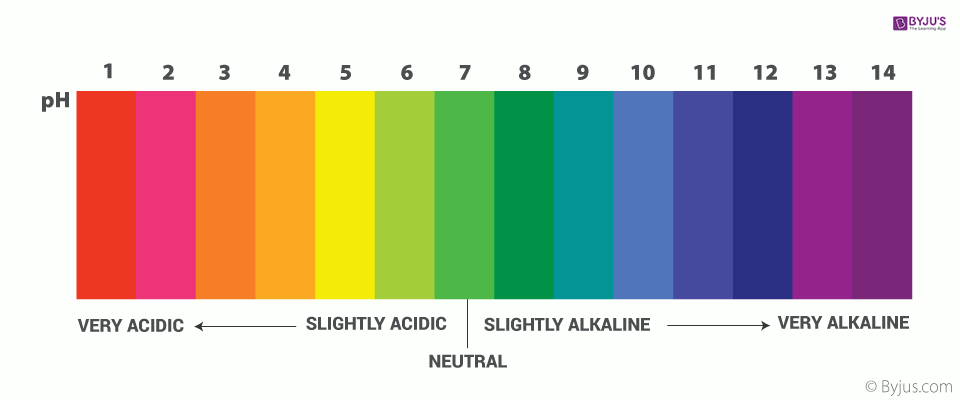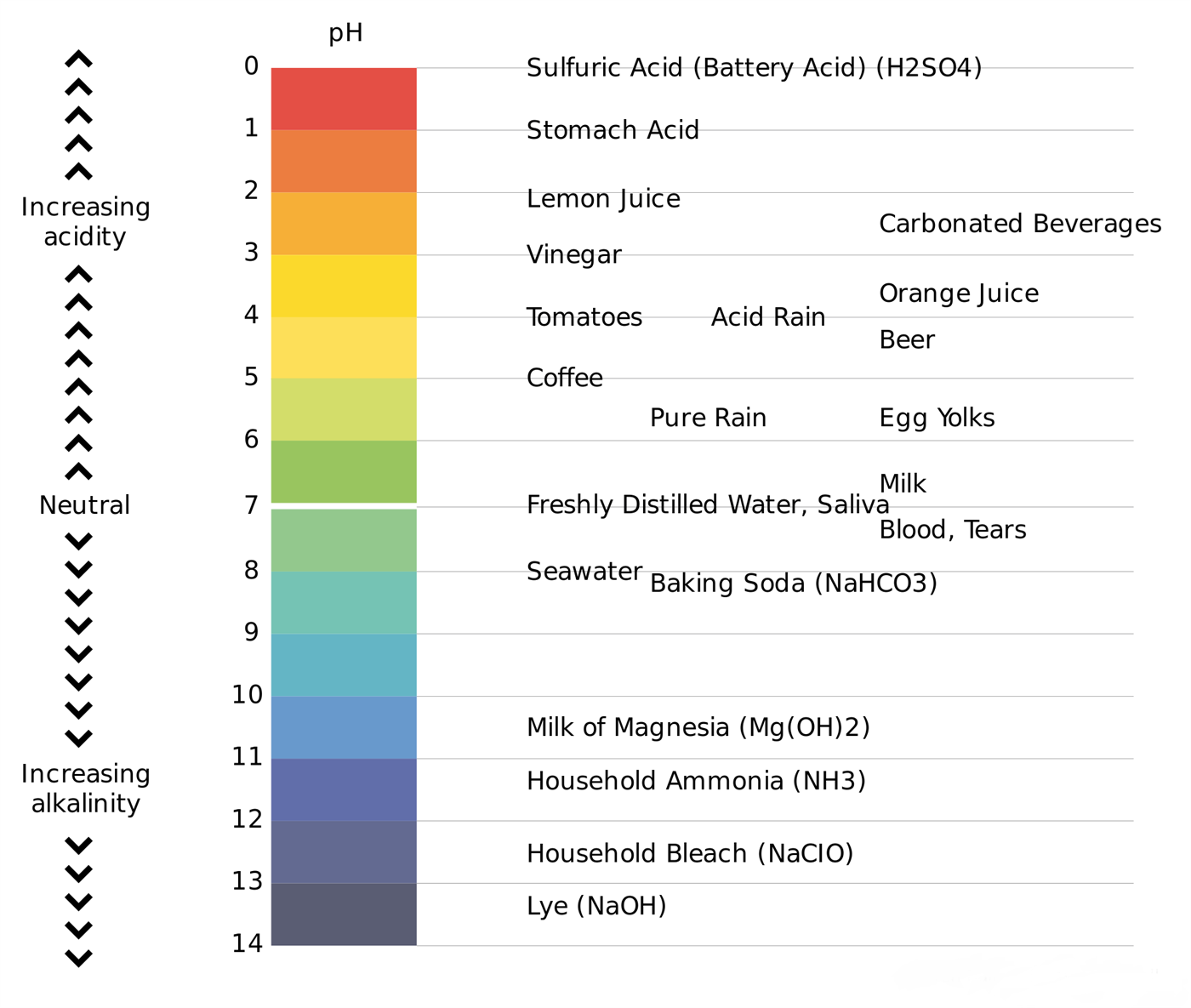# pH Of Acids And Bases

## What is pH?

### pH Definition

pH is defined as the negative logarithm of H+ ion concentration. Hence the meaning of the name pH is justified as the power of hydrogen.

We know that all the acids and bases do not react with the same chemical compound at the same rate. Some react very vigorously, some moderately while others show no reaction. To determine the strength of acids and bases quantitatively, we use a universal indicator which shows different colours at different concentrations of hydrogen ions in solution. Generally, the value of pH of acids and bases are used to quantitatively determine their strength.

## pH Chemistry

A pH scale is a tool for measuring acids and bases. The scale ranges from 0-14. Litmus paper is an indicator used to tell if a substance is an acid or a base. The colour of the paper matches up with the numbers on the pH scale to indicate what kind of substance is being tested. For example, Vinegar is an acid and measures 2.4 on the pH scale.

A healthy pH balance plays a significant role in your overall well-being, and doctors and scientists usually agree on this. The pH level, or possible level of hydrogen in your body, is determined by the food and type of drink you consume. The pH is the concentration of the hydrogen ions. This calculation is based on a pH scale.

The pH scale is logarithmic and shows the solution’s concentration of hydrogen ions inversely. More specifically, the pH of the solution is the negative logarithm to the base 10 of its hydrogen ion concentration in moles per litre.

The pH scale can be traced to a series of standard solutions whose pH is defined by international agreement. By calculating the potential difference between a hydrogen electrode and a standard electrode such as the silver chloride electrode, primary pH standard values are calculated using a concentration cell with transference.

A glass electrode and pH metre, or colour-changing indicator, may be used to measure the pH of aqueous solutions.pH of Acids and Bases – Red Litmus Test

## pH Abbreviation

pH may be seen as an abbreviation of the power of hydrogen-or, more fully, the concentration of hydrogen ion in a liquid.

Mathematical definition of pH is a little less intuitive but more useful in general. It states that the pH equals the negative logarithmic value of the concentration of hydrogen ion (H+)

pH = -log [H+]

A water source ‘s pH value is a function of its acidity, or alkalinity. The pH level is a function of the hydrogen atom activity, as the hydrogen activity is a reasonable indicator of the water’s acidity or alkalinity. As seen below, the pH scale ranges from 0 to 14 when 7.0 is neutral. It is said that water with a low pH is acidic and that water with a high pH is basic, or alkaline. Pure water should have a pH of 7.0 however, due to pollutants in the water, water supplies and precipitation appear to be slightly acidic.

In view of the defined scale, pH is a decided value, like the temperature. This means that the pH of water is not a physical parameter that can be measured either as a fixed, or in a quantity. Rather, it’s a nearby number of 0 and 14 that characterizes how acidic or basic a body of water is in a logarithmic scale. The smaller the amount, the more acidic the water will be. The higher the list, the more basic it is.

## Why does a Water Source Change pH?

Surface water usually has a pH value of 6.5 to 8.5 and groundwater appears to have a pH of 6.0 to 8.5. The pH of a source of water can naturally vary. Some types of rock and soil, such as limestone, can more effectively neutralize acid than other rock and soil types, such as granite.

Or, when large numbers of plants grow in a lake or river, when they die and decompose, they release carbon dioxide. A weak carbonic acid is produced when the carbon dioxide interacts with the water; this can then cause the water body to decrease its pH.

## With Increase in Temperature pH of Pure Water

As temperature increases, the degree of dissociation of water increases. Thus, the water dissociates to give more [H+], and hence its pH decreases. Temperature plays a significant role in pH measurements. As the temperature rises, molecular vibrations increase which results in the ability of water to ionize and form more hydrogen ions. As a result, the pH will drop.

pH decreases with an increase in temperature. In the case of pure water, there are always the same concentration of hydrogen ions and hydroxide ions and hence, the water is still neutral (even if its pH changes). At 100°C, a pH value of 6.14 is the new neutral point on the pH scale at this higher temperature.

## pH of Acids and Bases

The pH of a solution varies from 0 to 14.

• Solutions having a value of pH ranging from 0 to 7 on the pH scale are termed as acidic and the value of pH ranging from 7 to 14 on pH scale are known as basic solutions.
• Solutions having the value of pH equal to 7 on pH scale are known as neutral solutions.

Solutions having the value of pH equal to 0 are known to be strongly acidic solutions. Further, the acidity decreases as the value of pH increases from 0 to 7 whereas, solutions with the value of pH equal to 14 are termed as strongly basic solutions.

The basicity decreases as the value of pH decreases from 14 to 7. The strength of acids and bases depends on the number of H+ and OH ions produced. Acids furnishing more number of H+ ions are known to be strong acids and vice versa.

The degree of ionisation of acids and bases differs for different acids and bases. It helps in the determination of the strength of acids and bases. The strength of an acid depends on the concentration of hydronium ion (H3O+) too. With the help of the comparison between the concentration of hydronium ion and the hydroxyl ion, we can distinguish between acids and bases.

• For acidic solution: [H3O+] > [OH]
• For neutral solution: [H3O+] = [OH]
• For basic solution: [H3O+] < [OH]

## Limitations of pH Scale

pH value of a solution does not instantaneously give us an idea of the relative strength of the solution.

• pH is zero for 1N solution of strong acid.
• pH is negative for concentrations 2N, 3N, ION of strong acids.
• At higher concentrations, in place of pH Hammett acidity functions are used.

## pH ScalepH Scale

The concentration of hydronium ion is conveniently expressed on a logarithmic scale. This scale is known as pH scale. pH of acids and bases is defined as the negative logarithm (with base 10) of activity of hydrogen ion (H+).

## Recommended Videos## How to Calculate pH?

The activity has no units and is defined in terms of H+ ion concentration,

$$\begin{array}{l}a_{H^+}= [H^+{}]/mol \ L^{-1}\end{array}$$

Hence, the pH of a compound is given as,

$$\begin{array}{l}pH=-log\ a_{H^+} \end{array}$$

### Example

Thus, the pH of an acidic solution of HNO3 (10–3 M) = 3, a basic solution of KOH having [OH] =10–4M and [H3O+] =10–10 M will have a pH = 10. pH of acids is generally less than 7 whereas for bases it is greater than 7. At 298 K, ionic product of water, Kw can be given as:

Kw = [H3O+] [OH] = 10–14

Taking the negative logarithm of RHS and LHS, we deduce

–log Kw = – log ([H3O+] [OH])=   – log 10–14

=>pKw= – log [H3O+] – log [OH] = 14

=>pKw = pH + pOH = 14

The value of pKw controls the relative concentrations of hydrogen and hydroxyl ions as their product is always constant. pH of acids and bases can be roughly calculated with the help of pH scale while for accurate values we use pH meters.pH Example Chart

## pH Value and Nature of a Solution

• If [H+] > 10-7, pH is less than 7 and the solution is acidic.
• If [H+] = 10-7, pH is 7 and the solution is neutral.
• If [H+] < 10-7, pH is more than 7 and the solution is basic.

## Importance of pH

• Only a narrow range of pH change can be sustained by a living organism, any further change in pH can make the living difficult. For example: in the case of acid rain, the pH of water is less than 7. As it flows into a river, it lowers the pH of river water which makes the survival of aquatic life difficult.
• We know that our stomach contains hydrochloric acid which helps in the digestion of food. When the stomach produces too much of hydrochloric acid during indigestion, we feel a lot of pain and irritation. Hence, we generally use antacids or a mild base which increases the pH of the acidic stomach and thus decreases the pain.
• Bacteria present in our mouth sometimes lower the pH of our mouth by producing acids through degradation of the food particle. Hence, we are instructed to clean our mouths with toothpaste (which is generally basic) to prevent their decay by maintaining the pH.
• We experience a lot of pain in case of bee-sting as the bee injects the methanoic acid through its sting. Hence, we are generally advised to apply baking soda or other mild bases on the surface as it helps in maintaining the pH of the surface.

## Points to Remember on pH Scale

• pH of strong acid or base does not depend upon temperature.
• pH of weak acid decreases with increase in temperature due to increase in ionization.
• pH of weak base increases with increase in temperature due to increase in ionization or [OH-] ion concentration.

## Frequently Asked Questions – FAQs

Q1

### What is pH scale in chemistry?

The pH is a measure of the concentration of hydrogen ions, the acidity or alkalinity of a solution. The pH-scale is normally between 0 and 14. Aqueous solutions at 25 ° C with a pH of less than 7 are acidic and basic or alkaline solutions are those with a pH greater than 7.

Q2

### What is the best pH for the human body?

For our blood and body tissues the optimum pH is about 7.2. (The use of saliva and urine test strips will show a much lower pH level due to the protein present in the solution. A healthy body saliva and urine tests should have a pH of 6.6 to 6.8 approximately.) Acidosis is a condition of excess acidity in the blood and body tissue.

Q3

### What is pH full form?

PH stands for Hydrogen potentials. It refers to the concentration of the hydrogen ions in a solution. This is the indicator of a solution’s acidity or alkalinity. The pH value on a pH-scale varies from 0 to 14.

Q4

### Is pH of water important?

PH is a function of the acid / basic water content. Water with more free hydrogen ions is acidic and water with more free hydroxyl ions is basic. Because pH in the water can be changed by chemicals, pH is a significant indicator of water that is chemically changing.

Q5

### What happens if your pH is too high?

An increase in alkaline causes a rise in pH levels. When the acid levels are too high in the blood, it’s called acidosis. This is called alkalosis when the blood is too alkaline. A problem with the lungs is due to respiratory acidosis and alkalosis.

Q6

### What causes high pH in water?

The cause of the unbalanced pH is the soil, bedrock, or other underlying composition from which the water source comes. High alkaline water is a consequence of rocky areas with a lot of calcareous. It contains compounds of carbonate, bicarbonate, and hydroxide that dissolve and migrate with the water, increasing its pH.

Q7

### What is the pH of blood?

Humans have a typical pH range from 7.35 to 7.45. That means blood is of course very alkaline or normal. Your stomach acid, by contrast, has a pH of around 1.5 to 3.5. That makes it acidic.

Q8

### Who discovered the pH?

Exactly 100 years ago, Carlsberg ‘s director of chemistry, Søren Sørensen, developed a vital diagnostic tool for measuring acidity, thus helping to detect digestive , respiratory and metabolic disorders. The invention of Sørensen was the pH scale.

For detailed discussions on pH of acids and bases and its importance, Download BYJU’S – The Learning App.

Test your knowledge on ph of acids and bases